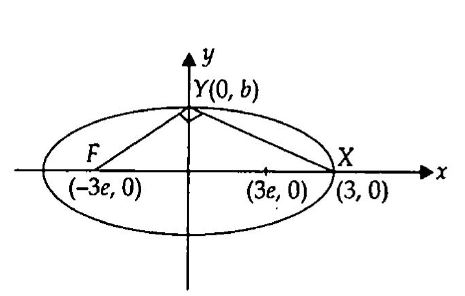# Q. Suppose X is one end of the major axis of the ellipse $\frac{x^2}{9} + \frac{y^2}{b^2} = 1$ (b < 3) and F is that focus which is farther from X. Let Y denote one end of the minor axis. If $FYX$ is a right angled triangle, then the length of the latus rectum of the ellipse is

COMEDK COMEDK 2005Conic Sections

Solution:

## $\Delta FYX$ is a right angle triangle.Slope of $FY\times$ Slope of $XY = - 1$ $\frac{0-b}{-3e -0} \cdot \frac{0-b}{3-0} = -1 \Rightarrow b^{2}= 9e$ We know, $e^{2} = 1 - \frac{b^{2}}{a^{2}} \Rightarrow \frac{b^{4}}{81} = 1-\frac{b^{2}}{9}$ $\Rightarrow b^{4} + 9b^{2} -81 = 0$ $\Rightarrow b^{2} = \frac{-9\pm \sqrt{81 +\left(4\times1\times81\right)}}{2\times1}$ $\Rightarrow b^{2} = \frac{-9\pm9\sqrt{5}}{2} \Rightarrow b^{2} = \frac{-9 + 9\sqrt{5}}{2}$ Length of latus rectum is $\frac{2b^{2}}{a}$ $= \frac{2\left(-9 +9\sqrt{5}\right)}{2\times3} = 3\left(\sqrt{5}-1\right)$

You must select option to get answer and solution

## 1. The function $f(x)=[x]$ where $[x]$ denotes the greatest integer not greater than $x,$ is...

KCET 2010 Continuity and Differentiability

## 2. The number of 4 digit numbers without repetition that can be formed using the digits 1, 2, 3, 4, 5, 6, 7 in which each number has two odd digits and two even digits is

KCET 2019 Permutations and Combinations

## 3. Let $P=[a_{ij}]$ be a $3\times3$ matrix and let $Q=[b_{ij}]$ where $b_{ij}=2^{i+j} a_{ij}$ for $1 \le i, j \le$.If the determinant of $P$ is $2$, then the determinant of the matrix $Q$ is

IIT JEE 2012 Determinants

## 4. The locus represented by $xy + yz = 0$ is

KCET 2018 Three Dimensional Geometry

## 5. If $P$ and $Q$ are symmetric matrices of the same order then $PQ - QP$ is

KCET 2019 Matrices

## 6. $\frac{1 -\tan^2 15^\circ}{1 + \tan^2 15^\circ} =$

COMEDK 2010 Trigonometric Functions

## 7. If $y =\sin^{-1} \left(\frac{5x+12 \sqrt{1 -x^{2}}}{13}\right)$ , then $\frac{dy}{dx} =$

COMEDK 2008 Continuity and Differentiability

## 8. If a complex number lies in the III quadrant. Find the quadrant in which its. conjugate lies.

COMEDK 2014 Complex Numbers and Quadratic Equations

## 9. If $(\overrightarrow{a} \times \overrightarrow{b})^2+(\overrightarrow{a}.\overrightarrow{b})^2=144$ and $|\overrightarrow{a}|$ then $|\overrightarrow{b}|$=

KCET 2012 Vector Algebra

## 10. The number of terms in the expansion of $(x^2 +y^2 )^{25} - (x^2 - y^2)^{25}$ after simplification is

KCET 2019 Binomial Theorem